# Chapter 3 Stoichiometry Chemical Stoichiometry The study of

• Slides: 36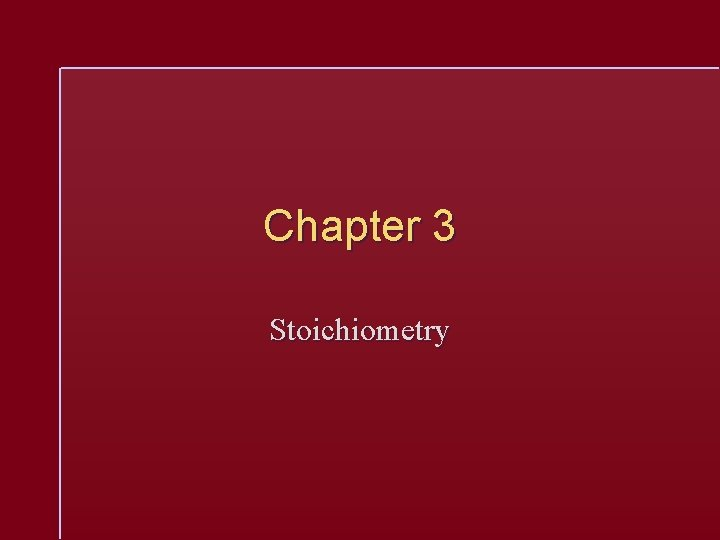Chapter 3 Stoichiometry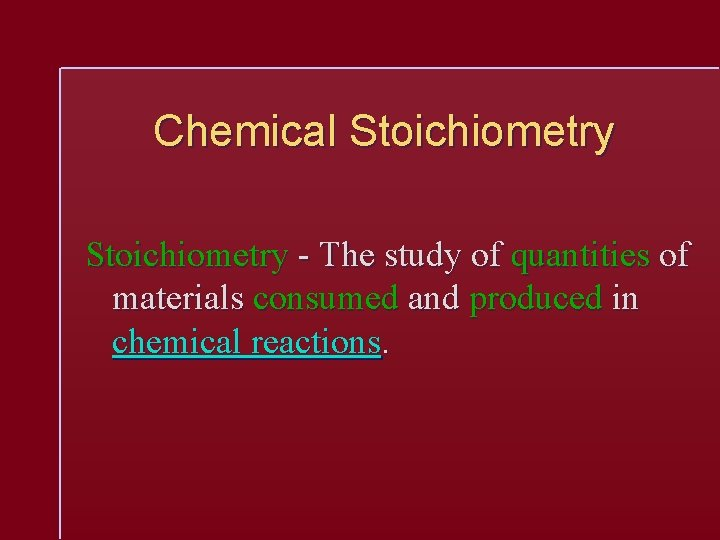Chemical Stoichiometry - The study of quantities of materials consumed and produced in chemical reactions.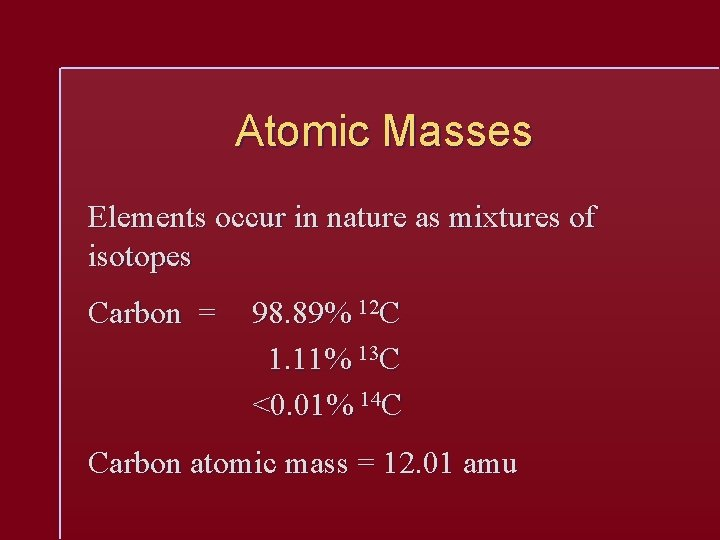Atomic Masses Elements occur in nature as mixtures of isotopes Carbon = 98. 89% 12 C 1. 11% 13 C <0. 01% 14 C Carbon atomic mass = 12. 01 amu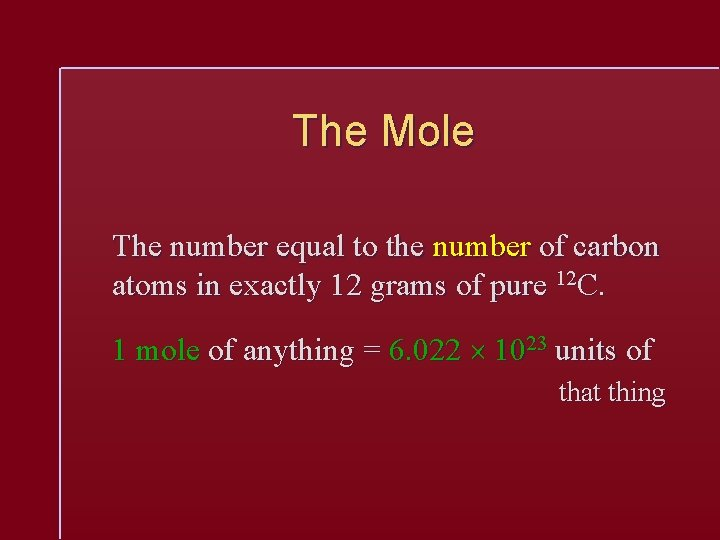The Mole The number equal to the number of carbon atoms in exactly 12 grams of pure 12 C. 1 mole of anything = 6. 022 1023 units of that thing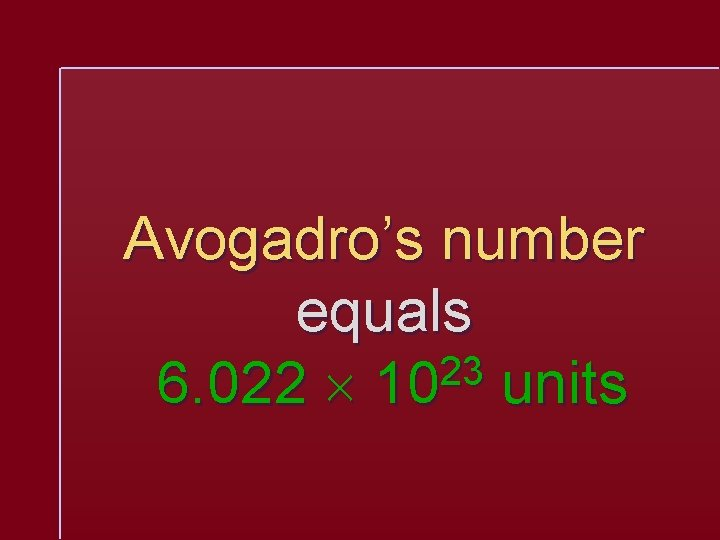Avogadro’s number equals 23 6. 022 10 units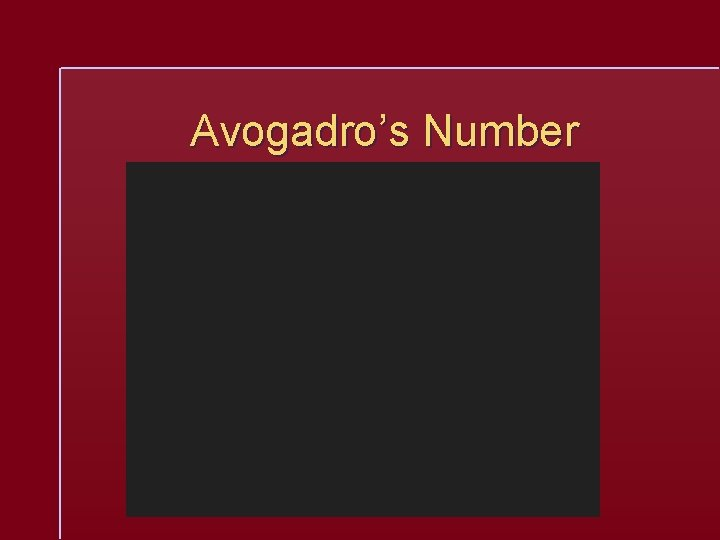Molar Mass A substance’s molar mass (molecular weight) is the mass in grams of one mole of the compound. CO 2 = 44. 01 grams per mole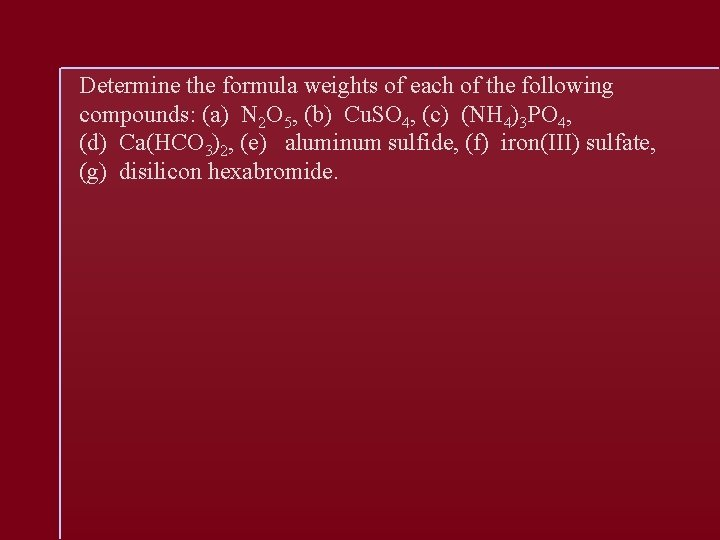Determine the formula weights of each of the following compounds: (a) N 2 O 5, (b) Cu. SO 4, (c) (NH 4)3 PO 4, (d) Ca(HCO 3)2, (e) aluminum sulfide, (f) iron(III) sulfate, (g) disilicon hexabromide.Calculate the following quantities: (a) mass, in grams, of 1. 906 × 10− 2 mol Bal 2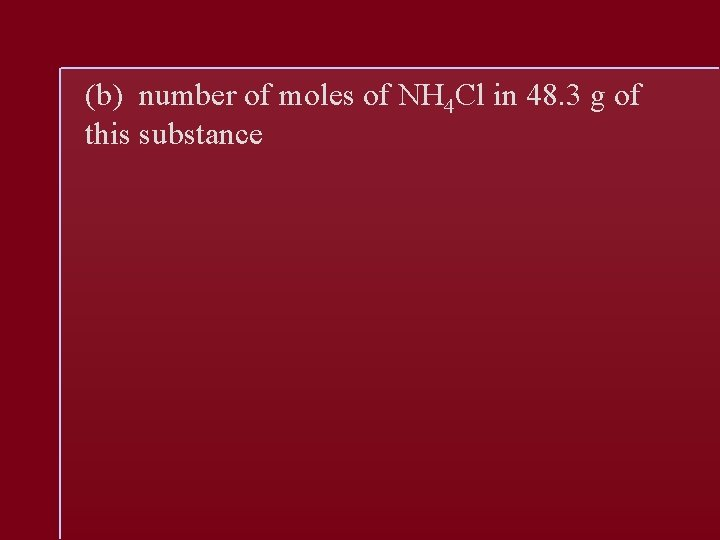(b) number of moles of NH 4 Cl in 48. 3 g of this substancec) number of molecules in 0. 05752 mol HCHO 2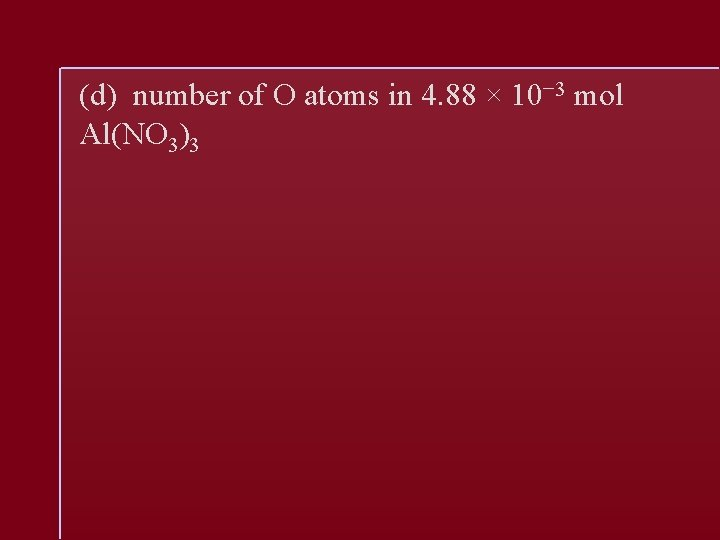(d) number of O atoms in 4. 88 × 10− 3 mol Al(NO 3)3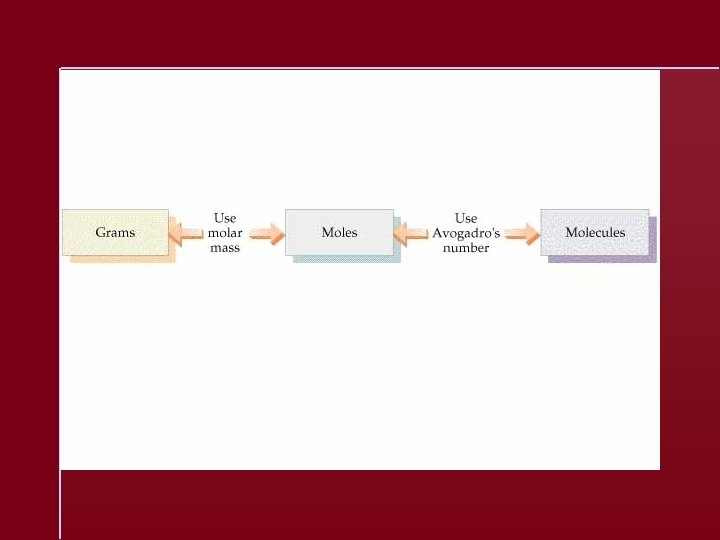The molecular formula of allicin, the compound responsible for the characteristic smell of garlic, is C 6 H 10 OS 2. (a) What is the molar mass of allicin? (b) How many moles of allicin are present in 5. 00 mg of this substance? (c) How many molecules of allicin are in 5. 00 mg of this substance? (d) How many S atoms are present in 5. 00 mg of allicin?Percent Composition Mass percent of an element: For iron in iron (III) oxide, (Fe 2 O 3)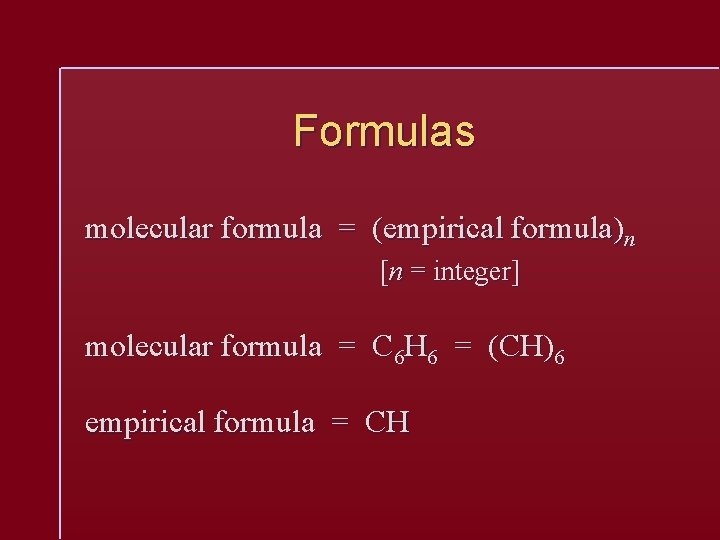Formulas molecular formula = (empirical formula)n [n = integer] molecular formula = C 6 H 6 = (CH)6 empirical formula = CHEmpirical Formula Determination 1. Base calculation on 100 grams of compound. 2. Determine moles of each element in 100 grams of compound. 3. Divide each value of moles by the smallest of the values. 4. Multiply each number by an integer to obtain all whole numbers.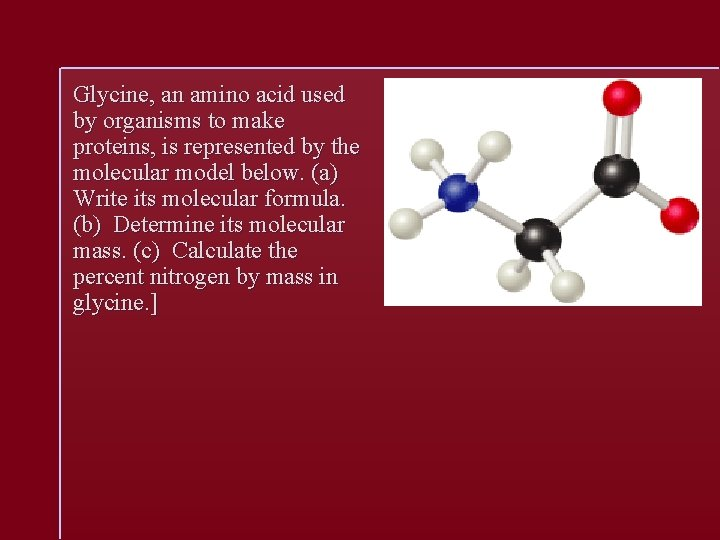Glycine, an amino acid used by organisms to make proteins, is represented by the molecular model below. (a) Write its molecular formula. (b) Determine its molecular mass. (c) Calculate the percent nitrogen by mass in glycine. ]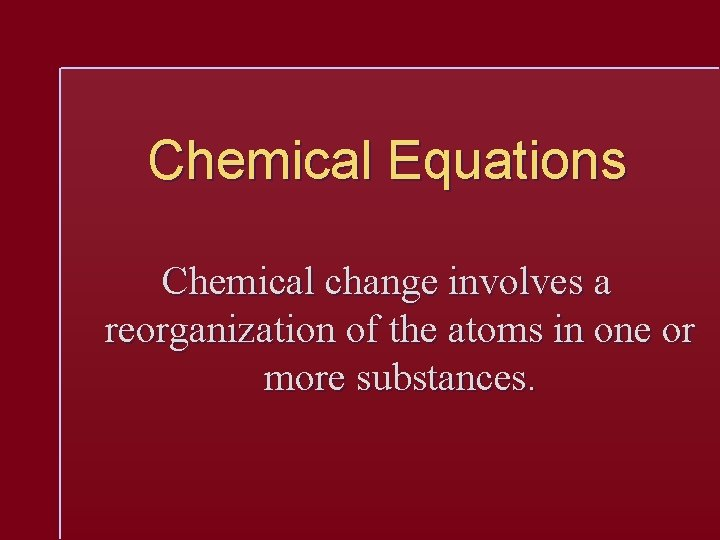Chemical Equations Chemical change involves a reorganization of the atoms in one or more substances.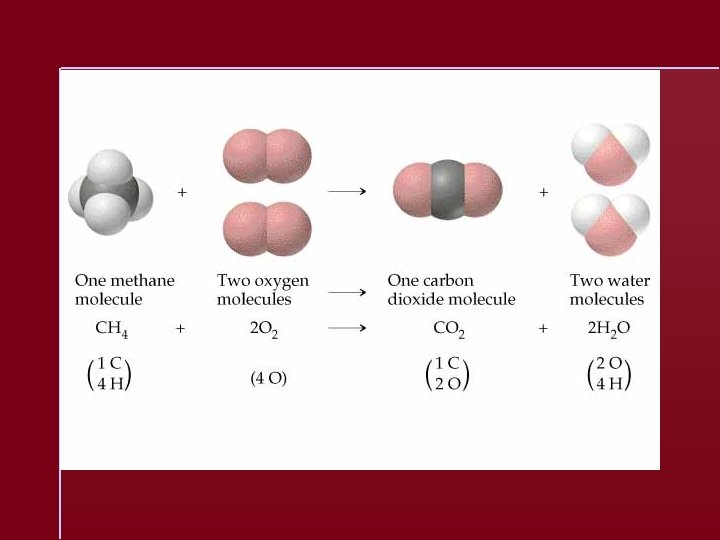Chemical Equation A representation of a chemical reaction: C 2 H 5 OH + 3 O 2 2 CO 2 + 3 H 2 O reactants productsChemical Equation C 2 H 5 OH + 3 O 2 2 CO 2 + 3 H 2 O The equation is balanced. 1 mole of ethanol reacts with 3 moles of oxygen to produce 2 moles of carbon dioxide and 3 moles of water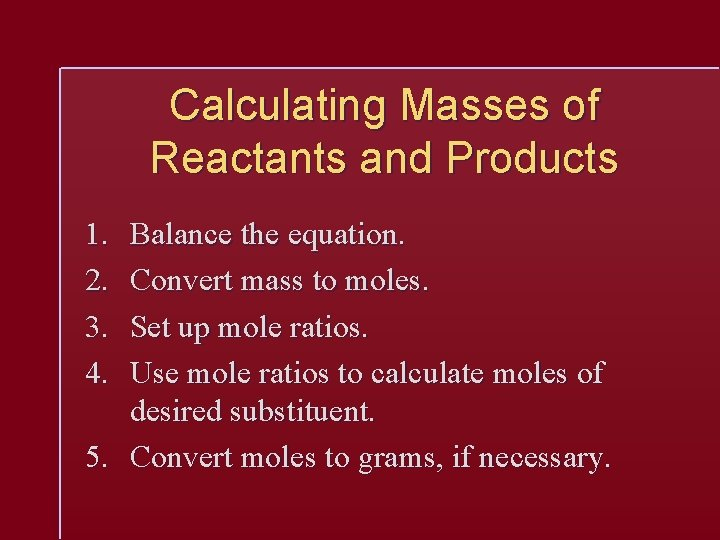Calculating Masses of Reactants and Products 1. 2. 3. 4. Balance the equation. Convert mass to moles. Set up mole ratios. Use mole ratios to calculate moles of desired substituent. 5. Convert moles to grams, if necessary.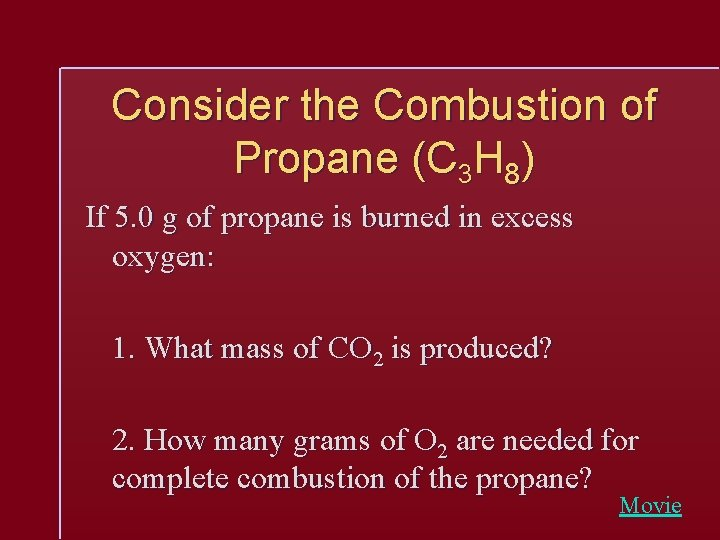Consider the Combustion of Propane (C 3 H 8) If 5. 0 g of propane is burned in excess oxygen: 1. What mass of CO 2 is produced? 2. How many grams of O 2 are needed for complete combustion of the propane? Movie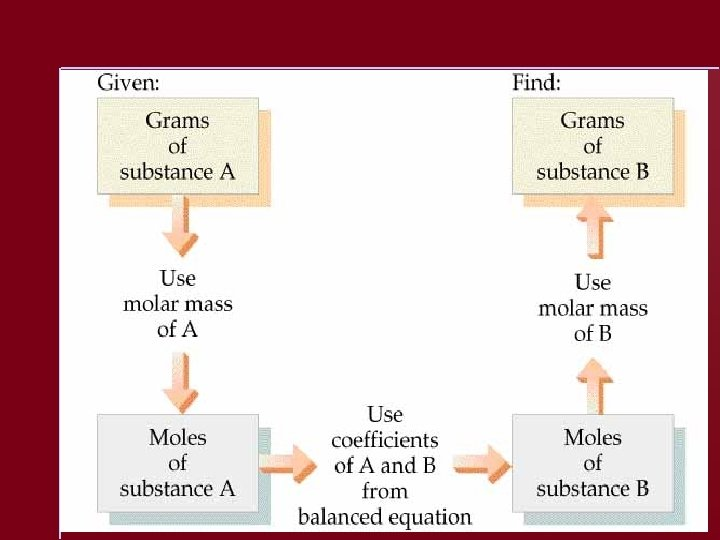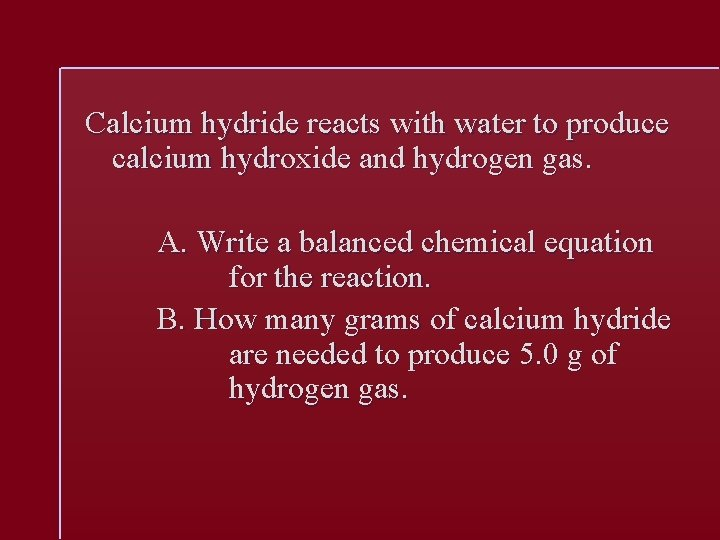Calcium hydride reacts with water to produce calcium hydroxide and hydrogen gas. A. Write a balanced chemical equation for the reaction. B. How many grams of calcium hydride are needed to produce 5. 0 g of hydrogen gas.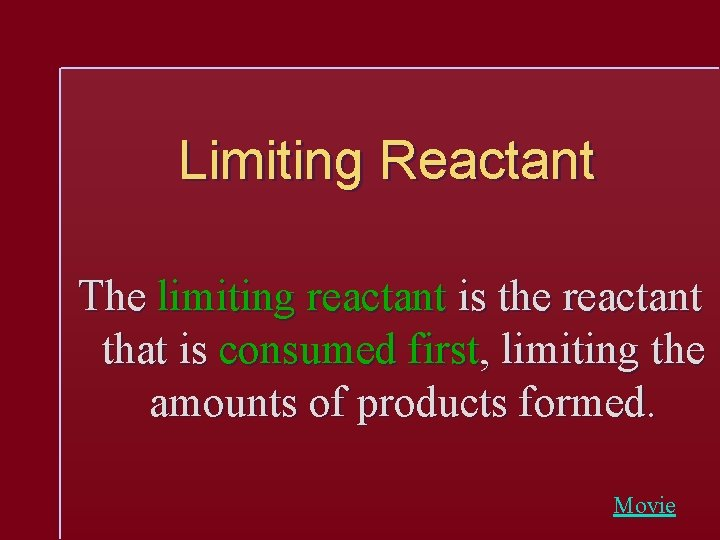Limiting Reactant The limiting reactant is the reactant that is consumed first, limiting the amounts of products formed. MovieSolving a Stoichiometry Problem 1. 2. 3. 4. Balance the equation. Convert masses to moles. Determine which reactant is limiting. Use moles of limiting reactant and mole ratios to find moles of desired product. 5. Convert from moles to grams.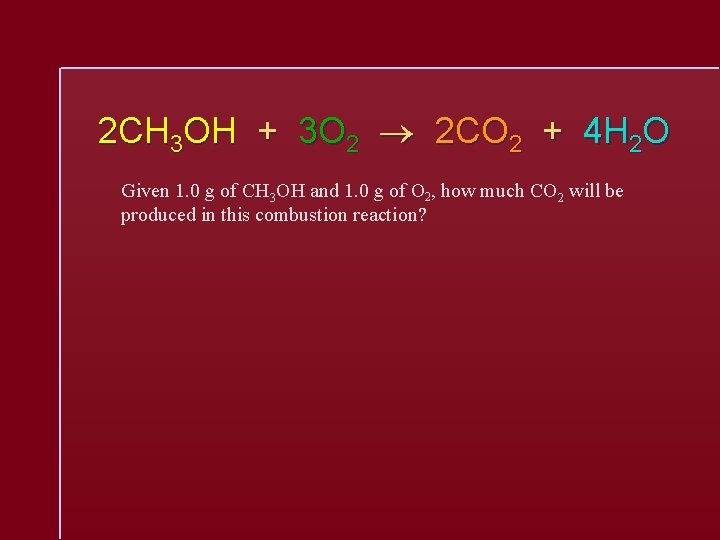2 CH 3 OH + 3 O 2 2 CO 2 + 4 H 2 O Given 1. 0 g of CH 3 OH and 1. 0 g of O 2, how much CO 2 will be produced in this combustion reaction?END